• This service has been inactive since 2010 as my new role in TM1 consulting.
• Please disregard any commercial offers as this website has been archived and transformed into a blog rather than a commercial website.

Live-updated Beta Calculation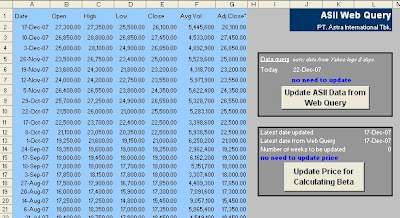Assume CAPM is applicable and works empirically without any doubt in the theory, we can then calculate a stock Beta dynamically over time as it represents the updated historical stock prices from the latest price. Hence, the stock Beta changes over time.

The process and the VBA coding are similar with the Live-updated Yield posted before.

This spreadsheet consists of 3 worksheets that dynamically calculates the beta of Astra stock returns (ASII.JK) against the Jakarta Composite index returns (^JKSE) as being updated over time by clicking the "Update" buttons. Thus, we can get the latest Beta of Astra stock.

The first worksheet is JKSE sheet that contains the recent updated market prices and the second one is ASII sheet for the recent updated stock prices. In these two sheets, there are two "Update" buttons that allow us to update the recent prices from web query and to record the recent prices into the Beta sheet (third worksheet).

The stock Beta is then calculated by simply finding the slope between the periodical stock (x axis) and market (y axis) returns.

Comprehensive Financial Planning

An Excel model to judge your prosperity and set your financial goal and objectives.Uni & Pension Plan... Hosted by eSnips

Value-at-Risk

Suppose you hold a portfolio like this.

Astra Int shares (ASII) with market value of IDR1,000,000,000.00
Indonesia Govt bonds (Indo-17) with market value of IDR1,000,000,000.00

Total market value of your portfolio is IDR2,000,000,000.00

You may wonder how much your portfolio value would be tomorrow in the worst-case scenario.

How do we get that value? Such value is known as Value-at-Risk (VaR).

The procedure:
1. Collect historical daily data for Astra share prices and calculate the daily return.
2. Collect historical daily date for Indonesia govt bonds yields, generate index and calculate the daily return.
3. Generate covariance matrix for share return and bond return.
4. Calculate portfolio variance and standard deviation. In Excel, it can be done using MMULT(MMULT(TRANSPOSE(value),covariancematrix),value)
5. Calculate VaR at 99% confidence level as 2.326 multiply by standard deviation.
6. Tomorrow's worst value = Today's value - VaR

From the example, I found tomorrow's worst case value is IDR1,937,346,708.11

Bond Price, Duration and ConvexityIn Feb 06 I submitted some short of article in Wikipedia about closed form formulas for bond duration and convexity. Below is the clone and hopefully it can be useful for those who may use the formulas for bond analysis modelling in Excel spreadsheets.

As far as I know, these have never been published on the web like this as I experienced some problem in finding them when doing some bond pricing project.

Closed-form formula:
A single arithmetic formula obtained to simplify an infinite sum in a general formula. The general formula of bond duration and bond convexity cannot be said closed-form as there is an infinite sum over the different time periods. Using a closed-form formula, a bond’s duration or convexity can be calculated at any point in its life time.

Bond duration closed-form formula (Richard Klotz):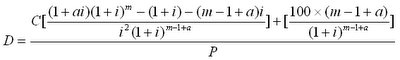C = coupon payment per period (half-year)
P = present value (price)
i = discount rate per period (half-year)
a = fraction of a period remaining until next coupon payment
m = number of coupon dates until maturity

Bond convexity closed-form formula (Blake and Orszag):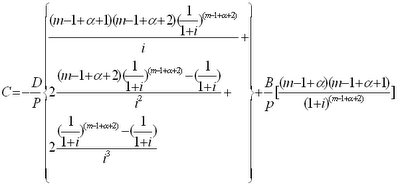D = coupon payment per period
P = present value (price)
B = face value
i = discount rate per period (half-year)
a = fraction of a period remaining until next coupon payment
m = number of coupon dates until maturity

Live-updated Yield

The purpose of this model is to provide a live-updated spreadsheet by automatically capturing the current data provided by a website via Web Query and then automatically recording the data into a master data sheet through a Bootstrapping method.

So, it's just two clicks away on our spreadsheet to update a time series graph to the current rate, automatically. It's similar when we are running a live-update for our antivirus software.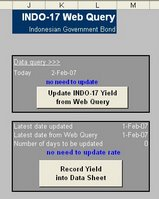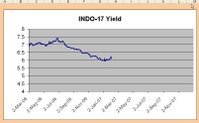The Worksheet

The Sheets

There are three sheets: INDO17, Data and Graph. "INDO17" is a sheet where the web query should go and where the two updating clicks are available. "Data" is a sheet where the bootstrapping process is running. "Graph" is only for visualisation.

Cell Naming

Cell naming is an important method as explained before in the previous post. There are several cells to be named in this worksheet and used for visual basic coding: INDO, INDOrow, INDOwebquery, nodays and updatelatestINDO.

To find which range is referred by a cell name, just go to the box on the top left, scroll the box and click the name. A range or cell will be shown.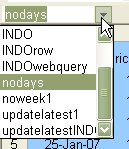• INDO: a range as the destination for the updated rate from 2-Mar-06 to 31-Dec-10 in "Data" sheet (B5:B1263).
• INDOrow: a cell showing a number of rows from 2-Mar-06 to the latest date has been updated in "Data" sheet (D3).
• INDOwebquery: a range as the source of data captured via web query in "INDO17" sheet (A2:D21).
• nodays: a cell showing a number of days need to be updated since the last updated date in "INDO17" sheet (M14).
• updatelatestINDO: a cell showing the next date needs to be updated in "Data" sheet (G4).

Visual Basic Coding

Web Query Update
This code is for the first button "Update INDO-17 Yield from Web Query". The webpage source used for web query is the INDO-17 yield data provided in the Central Bank of Indonesia website.

The code:

Sub dailyINDO()

Application.OnTime Now + TimeValue("12:00:00"), "updateINDO"

End Sub

Sub updateINDO()

Sheets("INDO17").Activate
Range("a1").Select
Selection.QueryTable.Refresh BackgroundQuery:=True
dailyINDO

End Sub

Recording Data through Bootstrapping
This code is for the second button "Record Yield into Data Sheet". The code is quite complicated. However, if we can follow the logic and as referring to the range names, this is a piece of cake for us.

The code:

Sub recordINDO()

For j = 1 To Range("nodays")
For i = 1 To Range("nodays")

If Sheets("Data").Range("updatelatestINDO") = Range("INDOwebquery").Cells(i, 1) Then
Range("INDOwebquery").Cells(i, 4).Copy

r = Sheets("Data").Range("INDOrow") + 1

Sheets("Data").Range("INDO").Cells(r).PasteSpecial Paste:=xlPasteValues
Calculate
End If

Next i
Next j

Sheets("Data").Select

End Sub

The meaning of the above code is:

For the loops as many as the number of days needs to be updated, Excel will copy each yield in the web query sheet and paste into the data sheet on each corresponding date. Where the corresponding date is found by referring the number of rows from the first date to the latest updated date plus 1 day after.

Piece of cake, aye?

Option for updating screen
You may insert this code to disable the visualisation of screen updating. Meaning, you can't see the price line moving forward as time moves.

Application.ScreenUpdating = False

The "INDOrow"

It seems the key problem here is to find the number of "INDOrow", a cell showing a number of rows from 2-Mar-06 to the latest date has been updated in "Data" sheet (D3).

The way is using a MATCH function to calculate the number of rows from 2-Mar-06 to the latest date as this formulae:
=MATCH("latest date",C5:C498,0)

Where the "latest date" is found by tagging a note in the column C as the latest date using IF formulae: =IF(AND(the next yield=0, the current yield >0),"latest date","").

The "updatelatestINDO
"

This is a cell showing the next date needs to be updated in "Data" sheet (G4) that can be found easily using an INDEX function:

=INDEX(\$A\$5:\$A\$498,INDOrow+1)

Recommendation and Notes

The title is for updating government bond yield, but the application can be also for updating other time series data such as foreign exchange rates or share prices.

I also open for any suggestions particularly for any more simple VBA codes.

Please let 2 days lag from Bank Indonesia website for current rate. It doesn't mean they are lazy to update, they just have a lot of other things to do.

Dynamic Company ValuationSince the key parameters in valuation model are mostly influenced by market behaviour, then it is important to update some relevant market data in regular basis to see how they affect the analysis. The relevant market data may include risk-free rate, stock price and market index that are able to indicate changes in value.

This model introduces manipulation of data by automatically changing some market data via the tool of external data import in Excel and some VBA simple codes for refreshing the Web Query. The main goal is to adjust calculation in Beta and WACC in the framework of company valuation and also for Option and Bond analysis.

In projecting financial statement, the historical data of the last two year operation is used to find the assumptions in the form of financial ratios that are normally corresponded to Sales. Then, the average of the last two year ratios can be used as the basic assumptions. Sales Growth ratio can be used as a look-up parameter in sensitivity analysis.

Sensitivity models are used by utilising two important tools in Excel, i.e., Data Tables and Graphs. Both tools are set to show visual changes in value after altering the growth ratio to some levels. This model also introduces sensitivity analysis of changes in financial ratios for the goal of predicting the possibility of bankruptcy. Traditional financial ratio techniques used are for Altman Bankruptcy model and Chesser Loan Surveillance model to be visually compared with the equity value and stock price resulted from the Free Cash Flow valuation.

The key analysis in this model is the Free Cash Flow valuation that has been as the important technique explained in the course and from the text. The method follows the that has been explained in the class using the No-Negative Cash and Debt as the Plugs. Some modification is tried to be applied by using Bonds as the plug, where the Bank Loans is calculated based on the bank loans to bonds ratio. The sum of both is the Long-term Debt.

Using bonds as the plug, the plan is to set of a Bond Portfolio for covering future financing in the projection. Then, the analysis refers to Net Present Value and Portfolio Duration that may anticipate in the future. As a bond issuer, the company should expect the lower value and duration.

Bond Portfolio AnalysisUsing the closed-form bond formula posted before, this bunch of spreadsheet is produced to value a bond at any point in its life time and do some analyses, as follows:
• Portfolio valuation, duration and convexity
• Derivatives valuation: FRA, Swap, Futures
• Risk governance: Value-at-Risk, Stress Testing and Scenario Analysis
• Hedging effectiveness
• Liquidity analysis

Remark: this portfolio is a liability/debt portfolio, NOT an asset/investment portfolio.

WACC Calculation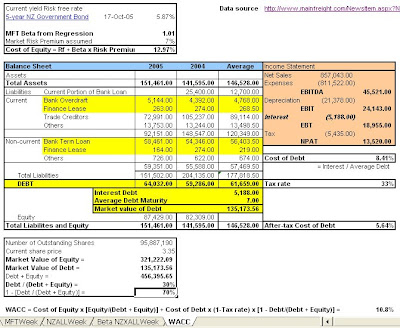This model is to estimate the weighted average cost of capital (WACC) of Mainfreight Limited (MFT), an NZX listed company.

Bear in mind, the debt and equity values should be estimated as the MARKET VALUES.

Some may just estimate the BETA, but in this model I calculated it based on the historical share prices. I used NZX ALL index as the market proxy and 5 year NZ Government Bond as the risk-free rate with risk premium assumed 7%.WACC Calculation.x... Hosted by eSnips

Share Valuation

This model is the share valuation of PT Astra International Tbk using Dividend Discount Model with assumed growth of 40%.

This should be the simplest way to value a share. By calculating the cost of equity as the discount factor, the projection of dividend cash flow is discounted to get the estimated value.

Seems similar with growth annuity valuation?ShareValue.xls Hosted by eSnips

Value-at-Risk

Suppose you hold a portfolio like this.

Astra Int shares (ASII) with market value of IDR1,000,000,000.00
Indonesia Govt bonds (Indo-17) with market value of IDR1,000,000,000.00

Total market value of your portfolio is IDR2,000,000,000.00

You may wonder how much your portfolio value would be tomorrow in the worst-case scenario.

How do we get that value? Such value is known as Value-at-Risk (VaR).

The procedure:
1. Collect historical daily data for Astra share prices and calculate the daily return.
2. Collect historical daily date for Indonesia govt bonds yields, generate index and calculate the daily return.
3. Generate covariance matrix for share return and bond return.
4. Calculate portfolio variance and standard deviation. In Excel, it can be done using MMULT(MMULT(TRANSPOSE(value),covariancematrix),value)
5. Calculate VaR at 99% confidence level as 2.326 multiply by standard deviation.
6. Tomorrow's worst value = Today's value - VaR

From the example, I found tomorrow's worst case value is IDR1,937,346,708.11

AstraWatch: week 29 Oct 07

PT Astra International Tbk (ASII)

Live-updated Value
Growth 16.00%
BETA 1.298713447
WACC 21.35%
Equity value (in IDR ,000,000,000) 42,757.86
Value per share (in IDR) 10,561.79